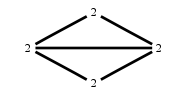# The Markov Bases Database

## G17_bin

The binary graph model of G17.
The binary matroid model of rank 2 on 4 binary random variables with added columns 01, 11.

It is a hierarchical model of 4 variables. The dimension of the model is 9.The cardinality of the statespace is 16.

### Properties of the Markov basis

 Markov degree 4 35

 degree # of gen. 2 4 4 31

The model has the following properties:

• All variables are binary.
• It is a graph model.
• The semigroup is normal.### FILES

 Markov basis: sufficient statistics matrix: G17_bin.mar (1.68 kb) G17_bin.mat (666 b) G17_bin.mod (43 b) G17_bin.tar.gz (5.49 kb)

Wrong or missing information? Write us an email.

page last update on November 15 2020. ->Contact